Documentation

# getLoopTransfer

Open-loop transfer function at specified point using `slLinearizer` or `slTuner` interface

## Syntax

``linsys = getLoopTransfer(s,pt)``
``linsys = getLoopTransfer(s,pt,sign)``
``linsys = getLoopTransfer(s,pt,temp_opening)``
``linsys = getLoopTransfer(s,pt,temp_opening,sign)``
``linsys = getLoopTransfer(___,mdl_index)``
``````[linsys,info] = getLoopTransfer(___)``````

## Description

example

````linsys = getLoopTransfer(s,pt)` returns the point-to-point open-loop transfer function at the specified analysis point for the model associated with the `slLinearizer` or `slTuner` interface, `s`.The software enforces all the permanent loop openings specified for `s` when it calculates `linsys`. If you configured either `s.Parameters`, or `s.OperatingPoints`, or both, `getLoopTransfer` performs multiple linearizations and returns an array of loop transfer functions.```

example

````linsys = getLoopTransfer(s,pt,sign)` specifies the feedback sign for computing the open-loop response. By default, `linsys` is the positive-feedback open-loop transfer function. Set `sign` to `-1` to compute the negative-feedback open-loop transfer function for applications that assume the negative-feedback definition of `linsys`. Many classical design and analysis techniques, such as the Nyquist or root locus design techniques, use the negative-feedback convention.The closed-loop sensitivity at `pt` is equal to `feedback(1,linsys,sign)`.```

example

````linsys = getLoopTransfer(s,pt,temp_opening)` considers additional, temporary, openings at the point specified by `temp_opening`. Use an opening, for example, to calculate the loop transfer function of an inner loop, measured at the plant input, with the outer loop open.```
````linsys = getLoopTransfer(s,pt,temp_opening,sign)` specifies temporary openings and the feedback sign.```

example

````linsys = getLoopTransfer(___,mdl_index)` returns a subset of the batch linearization results. `mdl_index` specifies the index of the linearizations of interest, in addition to any of the input arguments in previous syntaxes. Use this syntax for efficient linearization, when you want to obtain the loop transfer function for only a subset of the batch linearization results.```

example

``````[linsys,info] = getLoopTransfer(___)``` returns additional linearization information.```

## Examples

collapse all

Obtain the loop transfer function, calculated at `e`, for the `ex_scd_simple_fdbk` model.

Open the `ex_scd_simple_fdbk` model.

```mdl = 'ex_scd_simple_fdbk'; open_system(mdl); ```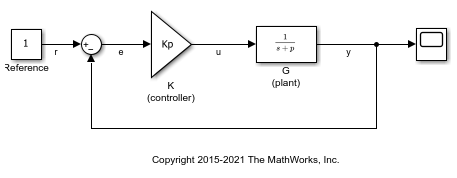In this model:

``

Create an `slLinearizer` interface for the model.

```sllin = slLinearizer(mdl); ```

To obtain the loop transfer function at `e`, add this point to `sllin` as an analysis point.

```addPoint(sllin,'e'); ```

Obtain the loop transfer function at `e`.

```sys = getLoopTransfer(sllin,'e'); tf(sys) ```
```ans = From input "e" to output "e": -3 ----- s + 5 Continuous-time transfer function. ```

The software adds a linearization output, breaks the loop, and adds a linearization input, `de`, at `e`.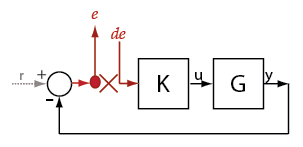`sys` is the transfer function from `de` to `e`. Because the software assumes positive-feedback, it returns `sys` as.

Obtain the negative-feedback loop transfer function, calculated at `e`, for the `ex_scd_simple_fdbk` model.

Open the `ex_scd_simple_fdbk` model.

```mdl = 'ex_scd_simple_fdbk'; open_system(mdl); ```In this model:

``

Create an `slLinearizer` interface for the model.

```sllin = slLinearizer(mdl); ```

To obtain the loop transfer function at `e`, add this point to `sllin` as an analysis point.

```addPoint(sllin,'e'); ```

Obtain the loop transfer function at `e`.

```sys = getLoopTransfer(sllin,'e',-1); tf(sys) ```
```ans = From input "e" to output "e": 3 ----- s + 5 Continuous-time transfer function. ```

The software adds a linearization output, breaks the loop, and adds a linearization input, `de`, at `e`.`sys` is the transfer function from `de` to `e`. Because the third input argument indicates negative-feedback, the software returns `sys` as.

Obtain the loop transfer function for the inner loop, calculated at `e2`, for the `scdcascade` model.

Open the `scdcascade` model.

```mdl = 'scdcascade'; open_system(mdl); ```Create an `slLinearizer` interface for the model.

```sllin = slLinearizer(mdl); ```

To calculate the loop transfer function for the inner loop, use the `e2` signal as the analysis point. To eliminate the effects of the outer loop, break the outer loop at `y1m`. Add these points to `sllin`.

```addPoint(sllin,{'e2','y1m'}); ```

Obtain the inner-loop loop transfer function at `e2`.

```sys = getLoopTransfer(sllin,'e2','y1m'); ```

Here, `'y1m'`, the third input argument, specifies a temporary loop opening. The software assumes positive-feedback when it calculates `sys`.

Suppose you batch linearize the `scdcascade` model for multiple transfer functions. For most linearizations, you vary the proportional (`Kp2`) and integral gain (`Ki2`) of the `C2` controller, in the 10% range. For this example, calculate the loop transfer function for the inner loop at `e2` for the maximum values of `Kp2` and `Ki2`.

Open the `scdcascade` model.

```mdl = 'scdcascade'; open_system(mdl); ```Create an `slLinearizer` interface for the model.

```sllin = slLinearizer(mdl); ```

Vary the proportional (`Kp2`) and integral gain (`Ki2`) of the `C2` controller in the 10% range.

```Kp2_range = linspace(0.9*Kp2,1.1*Kp2,3); Ki2_range = linspace(0.9*Ki2,1.1*Ki2,5); [Kp2_grid,Ki2_grid] = ndgrid(Kp2_range,Ki2_range); params(1).Name = 'Kp2'; params(1).Value = Kp2_grid; params(2).Name = 'Ki2'; params(2).Value = Ki2_grid; sllin.Parameters = params; ```

To calculate the loop transfer function for the inner loop, use the `e2` signal as the analysis point. To eliminate the effects of the outer loop, break the outer loop at `y1m`. Add these points to `sllin`.

```addPoint(sllin,{'e2','y1m'}); ```

Determine the index for the maximum values of `Ki2` and `Kp2`.

```mdl_index = params(1).Value == max(Kp2_range) & params(2).Value == max(Ki2_range); ```

Obtain the inner-loop loop transfer function at `e2`, with the outer loop open.

```sys = getLoopTransfer(sllin,'e2','y1m',-1,mdl_index); ```

The fourth input argument specifies negative-feedback for the loop transfer calculation.

```mdl = 'watertank'; open_system(mdl) ```Create a linearization option set, and set the `StoreOffsets` option.

```opt = linearizeOptions('StoreOffsets',true); ```

Create `slLinearizer` interface.

```sllin = slLinearizer(mdl,opt); ```

Add an analysis point at the tank output port.

```addPoint(sllin,'watertank/Water-Tank System'); ```

Calculate the loop transfer function at the analysis point, and obtain the corresponding linearization offsets.

```[sys,info] = getLoopTransfer(sllin,'watertank/Water-Tank System'); ```

View offsets.

```info.Offsets ```
```ans = struct with fields: x: [2x1 double] dx: [2x1 double] u: 1 y: 1 StateName: {2x1 cell} InputName: {'watertank/Water-Tank System'} OutputName: {'watertank/Water-Tank System'} Ts: 0 ```

## Input Arguments

collapse all

Interface to a Simulink model, specified as either an `slLinearizer` interface or an `slTuner` interface.

Analysis point signal name, specified as:

• Character vector or string — Analysis point signal name.

To determine the signal name associated with an analysis point, type `s`. The software displays the contents of `s` in the MATLAB® command window, including the analysis point signal names, block names, and port numbers. Suppose that an analysis point does not have a signal name, but only a block name and port number. You can specify `pt` as the block name. To use a point not in the list of analysis points for `s`, first add the point using `addPoint`.

You can specify `pt` as a uniquely matching portion of the full signal name or block name. Suppose that the full signal name of an analysis point is `'LoadTorque'`. You can specify `pt` as `'Torque'` as long as `'Torque'` is not a portion of the signal name for any other analysis point of `s`.

For example, `pt = 'y1m'`.

• Cell array of character vectors or string array — Specifies multiple analysis point names. For example, ```pt = {'y1m','y2m'}```.

To calculate `linsys`, the software adds a linearization output, followed by a loop break, and then a linearization input at `pt`. Consider the following model: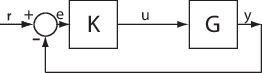Specify `pt` as `'u'`.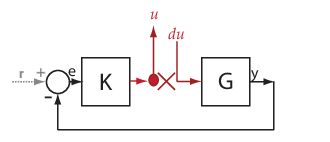The software computes `linsys` as the transfer function from `du` to `u`.

If you specify `pt` as multiple signals, for example `pt = {'u','y'}`, the software adds a linearization output, loop break, and a linearization input at each point.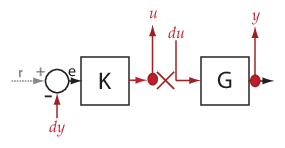`du` and `dy` are linearization inputs, and, `u` and `y` are linearization outputs. The software computes `linsys` as a MIMO transfer function with a transfer function from each linearization input to each linearization output.

Feedback sign, specified as one of the following values:

• `+1` (default) — `getLoopTransfer` returns the positive-feedback open-loop transfer function.

• `-1``getLoopTransfer` returns the negative-feedback open-loop transfer function. The negative-feedback transfer function is `-1` times the positive-feedback transfer function.

Temporary opening signal name, specified as:

• Character vector or string — Analysis point signal name.

`temp_opening` must specify an analysis point that is in the list of analysis points for `s`. To determine the signal name associated with an analysis point, type `s`. The software displays the contents of `s` in the MATLAB command window, including the analysis point signal names, block names, and port numbers. Suppose that an analysis point does not have a signal name, but only a block name and port number. You can specify `temp_opening` as the block name. To use a point not in the list of analysis points for `s`, first add the point using `addPoint`.

You can specify `temp_opening` as a uniquely matching portion of the full signal name or block name. Suppose that the full signal name of an analysis point is `'LoadTorque'`. You can specify `temp_opening` as `'Torque'` as long as `'Torque'` is not a portion of the signal name for any other analysis point of `s`.

For example, `temp_opening = 'y1m'`.

• Cell array of character vectors or string array — Specifies multiple analysis point names. For example, ```temp_opening = {'y1m','y2m'}```.

Index for linearizations of interest, specified as:

• Array of logical values — Logical array index of linearizations of interest. Suppose that you vary two parameters, `par1` and `par2`, and want to extract the linearization for the combination of ```par1 > 0.5``` and `par2 <= 5`. Use:

```params = s.Parameters; mdl_index = params(1).Value>0.5 & params(2).Value <= 5;```

The expression `params(1).Value>0.5 & params(2).Value<5` uses logical indexing and returns a logical array. This logical array is the same size as `params(1).Value` and `params(2).Value`. Each entry contains the logical evaluation of the expression for corresponding entries in `params(1).Value` and `params(2).Value`.

• Vector of positive integers — Linear index of linearizations of interest. Suppose that you vary two parameters, `par1` and `par2`, and want to extract the linearization for the combination of ```par1 > 0.5``` and `par2 <= 5`. Use:

```params = s.Parameters; mdl_index = find(params(1).Value>0.5 & params(2).Value <= 5);```

The expression `params(1).Value>0.5 & params(2).Value<5` returns a logical array. `find` returns the linear index of every true entry in the logical array

## Output Arguments

collapse all

Point-to-point open-loop transfer function, returned as described in the following:

• If you did not configure `s.Parameters` and `s.OperatingPoints`, the software calculates `linsys` using the default model parameter values. The software uses the model initial conditions as the linearization operating point. `linsys` is returned as a state-space model.

• If you configured `s.Parameters` only, the software computes a linearization for each parameter grid point. `linsys` is returned as a state-space model array of the same size as the parameter grid.

• If you configured `s.OperatingPoints` only, the software computes a linearization for each specified operating point. `linsys` is returned as a state-space model array of the same size as `s.OperatingPoints`.

• If you configured `s.Parameters` and specified `s.OperatingPoints` as a single operating point, the software computes a linearization for each parameter grid point. The software uses the specified operating point as the linearization operating point. `linsys` is returned as a state-space model array of the same size as the parameter grid.

• If you configured `s.Parameters` and specified `s.OperatingPoints` as multiple operating point objects, the software computes a linearization for each parameter grid point. The software requires that `s.OperatingPoints` is the same size as the parameter grid specified by `s.Parameters`. The software computes each linearization using corresponding operating points and parameter grid points. `linsys` is returned as a state-space model array of the same size as the parameter grid.

• If you configured `s.Parameters` and specified `s.OperatingPoints` as multiple simulation snapshot times, the software simulates and linearizes the model for each snapshot time and parameter grid point combination. Suppose that you specify a parameter grid of size `p` and `N` snapshot times. `linsys` is returned as a state-space model array of size `N`-by-`p`.

Linearization information, returned as a structure with the following fields:

Linearization offsets, returned as `[]` if `s.Options.StoreOffsets` is `false`. Otherwise, `Offsets` is returned as one of the following:

• If `linsys` is a single state-space model, then `Offsets` is a structure.

• If `linsys` is an array of state-space models, then `Offsets` is a structure array with the same dimensions as `linsys`.

Each offset structure has the following fields:

FieldDescription
`x`State offsets used for linearization, returned as a column vector of length nx, where nx is the number of states in `linsys`.
`y`Output offsets used for linearization, returned as a column vector of length ny, where ny is the number of outputs in `linsys`.
`u`Input offsets used for linearization, returned as a column vector of length nu, where nu is the number of inputs in `linsys`.
`dx`Derivative offsets for continuous time systems or updated state values for discrete-time systems, returned as a column vector of length nx.
`StateName`State names, returned as a cell array that contains nx elements that match the names in `linsys.StateName`.
`InputName`Input names, returned as a cell array that contains nu elements that match the names in `linsys.InputName`.
`OutputName`Output names, returned as a cell array that contains ny elements that match the names in `linsys.OutputName`.
`Ts`Sample time of the linearized system, returned as a scalar that matches the sample time in `linsys.Ts`. For continuous-time systems, `Ts` is `0`.

If `Offsets` is a structure array, you can configure an LPV System block using the offsets. To do so, first convert them to the required format using `getOffsetsForLPV`. For an example, see Approximating Nonlinear Behavior Using an Array of LTI Systems.

Linearization diagnostic information, returned as `[]` if `s.Options.StoreAdvisor` is `false`. Otherwise, `Advisor` is returned as one of the following:

`LinearizationAdvisor` objects store linearization diagnostic information for individual linearized blocks. For an example of troubleshooting linearization results using a `LinearizationAdvisor` object, see Troubleshoot Linearization Results at Command Line.

collapse all

### Loop Transfer Function

The loop transfer function at a point is the point-to-point open-loop transfer function from an additive disturbance at a point to a measurement at the same point.

To compute the loop transfer function at an analysis point, `x`, the software adds a linearization output, inserts a loop break, and adds a linearization input, `dx`. The software computes the transfer function from `dx` to `x`, which is equal to the loop transfer function at `x`.

Analysis Point in Simulink ModelHow `getLoopTransfer` Interprets Analysis Point Loop Transfer Function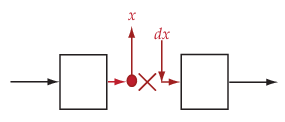Transfer function from `dx` to `x`

For example, consider the following model where you compute the loop transfer function at `e`: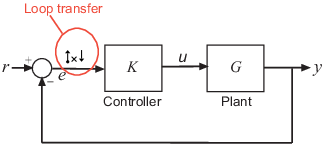Here, at `e`, the software adds a linearization output, inserts a loop break, and adds a linearization input, `de`. The loop transfer function at `e`, L, is the transfer function from `de` to `e`. L is calculated as follows:

`$e=\underset{L}{\underbrace{-GK}}de.$`

To compute -KG, use `u` as the analysis point for `getLoopTransfer`.

The software does not modify the Simulink model when it computes the loop transfer function.

### Analysis Points

Analysis points, used by the `slLinearizer` and `slTuner` interfaces, identify locations within a model that are relevant for linear analysis and control system tuning. You use analysis points as inputs to the linearization commands, such as `getIOTransfer`, `getLoopTransfer`, `getSensitivity`, and `getCompSensitivity`. As inputs to the linearization commands, analysis points can specify any open-loop or closed-loop transfer function in a model. You can also use analysis points to specify design requirements when tuning control systems using commands such as `systune`.

Location refers to a specific block output port within a model or to a bus element in such an output port. For convenience, you can use the name of the signal that originates from this port to refer to an analysis point.

You can add analysis points to an `slLinearizer` or `slTuner` interface, `s`, when you create the interface. For example:

`s = slLinearizer('scdcascade',{'u1','y1'});`

Alternatively, you can use the `addPoint` command.

To view all the analysis points of `s`, type `s` at the command prompt to display the interface contents. For each analysis point of `s`, the display includes the block name and port number and the name of the signal that originates at this point. You can also programmatically obtain a list of all the analysis points using `getPoints`.

For more information about how you can use analysis points, see Mark Signals of Interest for Control System Analysis and Design and Mark Signals of Interest for Batch Linearization.

### Permanent Openings

Permanent openings, used by the `slLinearizer` and `slTuner` interfaces, identify locations within a model where the software breaks the signal flow. The software enforces these openings for linearization and tuning. Use permanent openings to isolate a specific model component. Suppose that you have a large-scale model capturing aircraft dynamics and you want to perform linear analysis on the airframe only. You can use permanent openings to exclude all other components of the model. Another example is when you have cascaded loops within your model and you want to analyze a specific loop.

Location refers to a specific block output port within a model. For convenience, you can use the name of the signal that originates from this port to refer to an opening.

You can add permanent openings to an `slLinearizer` or `slTuner` interface, `s`, when you create the interface or by using the `addOpening` command. To remove a location from the list of permanent openings, use the `removeOpening` command.

To view all the openings of `s`, type `s` at the command prompt to display the interface contents. For each permanent opening of `s`, the display includes the block name and port number and the name of the signal that originates at this location. You can also programmatically obtain a list of all the permanent loop openings using `getOpenings`.

#### Introduced in R2013b